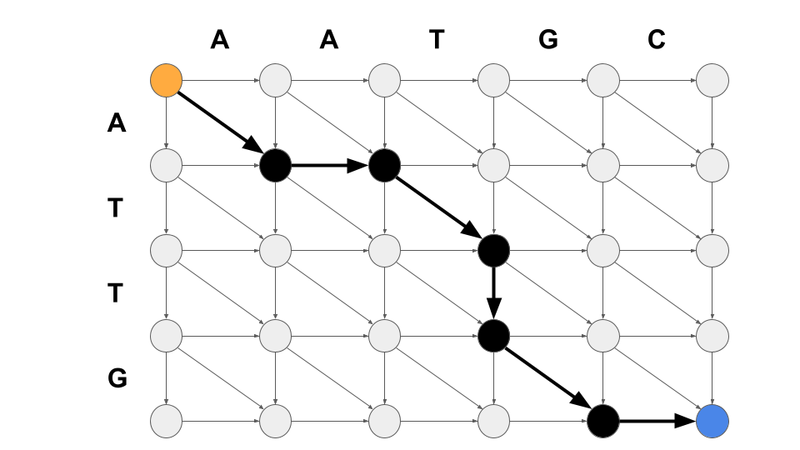# Complexity Explorer Santa Few Institute## Computation in Complex Systems (Spring 2022)

This course is no longer in session.

• Course Introduction
• Two Kinds of Paths
• Polynomials vs. Exponentials
• Divide and Conquer
• Big O and All that
• When the details don't matter
• Exam
• Divide and Conquer Redux
• Dynamic Programming
• Greedy Algorithms
• Reductions and Translations
• Lessons So Far
• The Best of All Possible Algorithms
• Complexity Wrap-Up
• Exam
• Finding versus Checking
• Circuits and Formulas
• More NP-complete Problems
• P versus NP Problem
• Existence and Nonexistence
• Above and Beyond
• Exam
• Real World Problems
• Phase Transitions
• Random Problems
• Solvability Threshold
• Modeling Differential Equations
• Landscapes, Clustering, Freezing, and Hardness
• Exam
• Building Blocks: Recursive Functions
• Building Blocks: Partial Recursive Functions
• λ Calculus
• Turing Machines
• The Halting Problem
• The Grand Unified Theory of Computation
• The Analytical Engine
• Cellular Automata
• Tile-Based Computation
• Dynamical Systems
• Exam
• More from Cris Moore
• Other ComplexityExplorer resources

#### 2.9 Quiz 2 (self-assessment) » Quiz 2: Explanation

Q1. What type of algorithm can efficiently find the minimum spanning tree below? The weights of the edges correspond to Euclidean distances.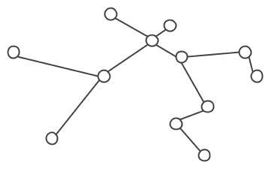(A) Recursive

(B) Greedy

(C) Dynamic programming

(D) Brute force

Explanation: From lecture 2.3, the minimum spanning tree can be efficiently solved using a greedy algorithm by searching the set of remaining nodes and iteratively adding the two nodes separated by the smallest distance. See lecture 2.3 [3:10] for Cris’s laid-out proof by contradiction.

Dynamic programming and recursive algorithms work well for problems that can be easily split into sub-problems (lecture 2.2), with recursion working especially well for problems with a trivial base case. Brute force algorithms are necessary for NP-complete and similar problems. However, none of these algorithms fit this particuarly spanning tree problem.

Q2. What type of algorithm can efficiently find the maximum weighted path from the top to the bottom of the triangle below? The path weight corresponds to the sum of the connected nodes. The possible path edges are indicated.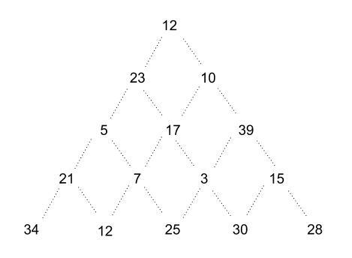(A) Recursive

(B) Greedy

(C) Dynamic programming

(D) Brute force

Explanation: Dynamic programming occurs when a problem is broken down into subroutines, with intermediate values stored during the execution of the problem. Here, dynamic programming can be used to store the maximum weight of each sub-tree, allowing for an easy determination of the maximally weighted path. This algorithm in action is shown below.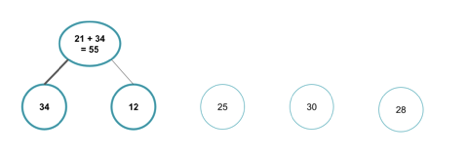Step 1: For each node of depth n-1, the maximal path weight is calculated. Here, the (21) node’s maximally weighted path includes the (34) node, for a total of 55. This value is stored in place of 21.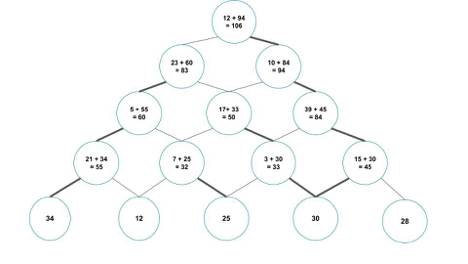Step 2 & 3: Repeat this algorithm for each subtree, each time using the stored value. The maximally weighted path is the only path which connects the root node to a leaf - in this case, [12, 10, 39, 15, 30).

This is similar to recursion, except for the storage of the sub-tree’s maximum value at each step. Recursion does not include this storage. Greedy programming would not be able to solve this problem, as it would start from the root node and always choose the highest value available for a result of [12, 23, 17, 7, 25]. Brute force will solve this problem, but not as efficiently as dynamic programming.

Q3. If problem M can be solved, and if problem M is reducible to problem N, which of the following are true of problem N?

(A) Problem N is solvable.

(B) Problem N is easier than problem M.

(C) Problem N is at least as hard as problem M.

(D) Problem N is reducible to problem M.

(D) None of the above.

Explanation: The term “reducible” here is a transformation from one problem into another (lecture 2.5). Specifically, if problem M is reducible to problem N, then if there exists an algorithm that solves N, that algorithm can be used to solve M, and M is not harder than N.

Problem N is therefore “at least as general” as problem M, and if N is easy, then so is M.

This does not mean N is solvable, or that N is easier than M (or, really, that N is "easy" at all) or N is reducible. All we know about N is that it is at least as hard as M.

Q4. The next series of questions relates to the binary trees A, B and C below.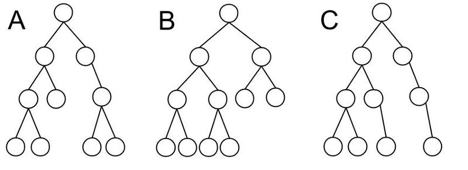Q4 (A): Which of these trees is most efficient?

(A) A

(B) B

(C) C

(D) A and B equally

(E) B and C equally

(F) A, B and C equally

Explanation: While all three trees are binary trees in that they have at most two children attached to each node, only B is a balanced tree. That means for a tree with an odd number of nodes, every node except the leaves have 2 children. For an even number of nodes, all nodes except 1 will have 2 children, and that node will be one level above the leaves. The performance of a balanced tree is exactly log(n), with n being the number of nodes.

The other trees are not balanced - the rightmost node below the root only has one child in both A and C. Therefore, their performance is greater than log(n), since at one point (that rightmost node below the root), there will not be a choice between two children.

For further reading, this Medium article has a number of good descriptions and drawings.

Q4 (B): How many leaves does tree A have?

(A) 3

(B) 4

(C) 5

(D) 6

(E) 10

Explanation: A leaf node is any node which does not have a sub-tree structure. That is, they do not have any children. A has 5 such nodes, shown in blue below.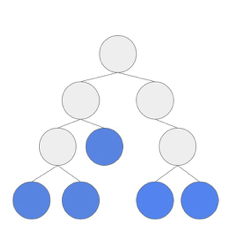Q4 (C): How many children does the root of tree B have?

(A) 2

(B) 4

(C) 6

(D) 8

(E) 10

Explanation: Since B is a balanced binary tree (see part (a) ), each node can only have at most two children. The root (or top) node fulfills the maximum number of children, highlighted in green below.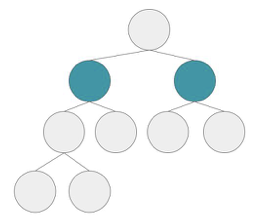Q4 (D): What is the height of tree C?

(A) 3

(B) 4

(C) 5

(D) 7

(E) 9

Explanation: The height of a tree is the maximum number of branching occurrences. For tree C (and the others), there are a maximum of three branching occurrences. The height can also be the number of “layers” of the tree, excluding the root node.

Q5. Which of the problems below could be solved with maximum flow?

(A) Delivering crates of cookies from a central factory to two hundred stores, with each delivery route i having a capacity to carry ni boxes

(B) Piping water from a well to twelve houses in a village, with each link of pipe i having a capacity to carry vi liters per minute.

(C) Matching players from two backgammon clubs, each with n players, against each other such that players will not play against the players k that they have already played.

(D) All of these.

(E) None of these.

Explanation: The maximum flow algorithm can be applied to a wide range of problems. Options (a) & (b) are both traditional capacity problems, while option (c), a bipartite matching problem, is reducible to the maximum flow problem (see lecture 2.5). For an alternative source, as well as a discussion on the BigO of this reduction, check out this lecture from the University of Maryland.

Q6. What is the factor of n that determines BigO for the following functions?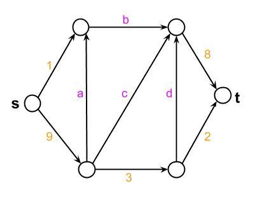Q6 (A): Which combination of capacities for edges a, b, c, d allows maximum flow (10) from source (s) to sink/target (t)?

(A) a=3 b=3 c=3 d=1

(B) a=3 b=4 c=3 d=1

(C) a=3 b=5 c=2 d=0

(D) a=6 b=6 c=0 d=1

Explanation: Only option (b) allows for the top-right node to hold 8 units, allowing for a full 11 units to reach node t. All others reduce the capacity of this top-right node by 1 or 2 units (see images below representing each option).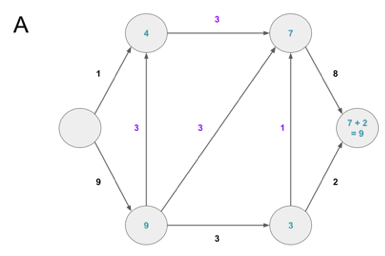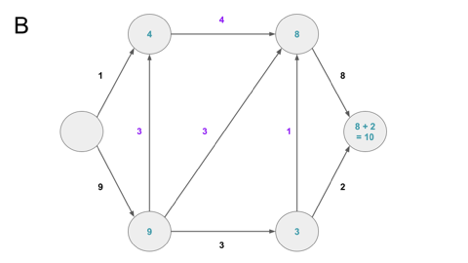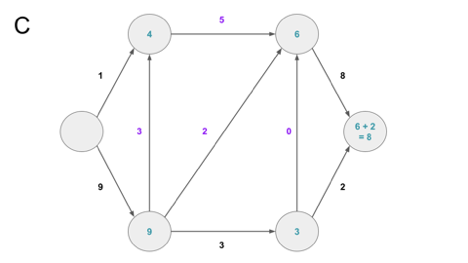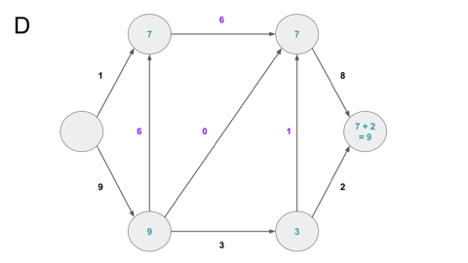Q6 (B): What is the maximum capacity from source (s) to sink/target (t)?

(A) 9

(B) 10

(C) 11

(D) 12

(E) 13

Explanation: Due to the limitation of the top horizontal edge, the maximum value which can be transferred to the top-right node is 6. Therefore, only 6 units can be transferred to the target node from the top node, while 5 may be transferred from the bottom node. Therefore, 11 units can access the sink at a single time. See the animation below for a visual representation of this process (note: the transfer between the top right and lower right nodes is ignored here, as it does not affect the final value).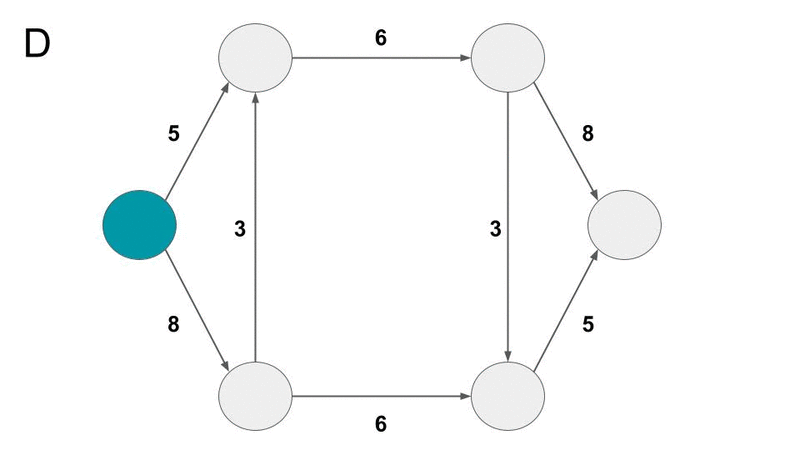Q7. The next questions pertain to the pseudocode below for calculating the nth index in the Fibonacci sequence.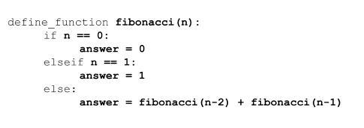Q7 (A): What type of algorithm is described by this approach?

(A) Recursive

(B) Dynamic programming

(C) Greedy

(D) Brute force

Explanation: A recursive function is one which breaks down a problem into sub-problems by iteratively calling itself. Recursive functions appear in many situations (a nested Russian Doll is one such example), and in computer science, a recursive function is distinguished by the fact it calls itself. There are one or more base cases, where the algorithm terminates. In this case, the cases where n=0 or n=1 will cause the program to end.

Q7 (B): What is its BigO class?

(A) O(1) = constant

(B) O(n) = linear

(C) O(n log n) = logarithmic

(D) O(n^C) = polynomial

(E) O(x^n) = exponential

Explanation: As n increases, the number of computational steps increases exponentially. A proof is below.

The definition of the Fibonacci sequence is:The characteristic equation (solvingfor, where) is:Using the quadratic forumla, we find:Note: We can pick one of the two values for x, since the complexity of the equation will be the same regardless of which value is chosen. Here, we choose the 1st value, bolded above.

Substitutingback into the original, we get:Converting to BigO notation through removing lower-order terms and multiples, we get:Solving the equation, we arrive at:Therefore, this recursive definition of fibonacci is exponential.

As an interesting aside, this code increases exponentially with X = ~1.6, which leads to the computational time necessary to calculate a fibonacci number (using recursion) being very close to the actual fibonacci number. Check out page 5 of this MIT lecture for a more detailed explanation of this.

Q8. The next questions pertain to the pseudocode below for calculating the nth index in the Fibonacci sequence.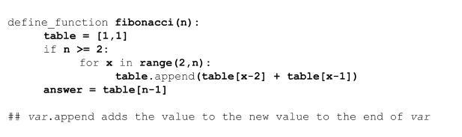Q8 (A): What type of algorithm is described by this approach?

(A) Recursive

(B) Dynamic programming

(C) Greedy

(D) Brute Force

Explanation: In contrast to the other algorithms listed, dynamic programming saves data as the algorithm progresses. Here, the table list utilizes previously calculated values to calculate the fibonacci value at x in the line table.append(table[x-2] + table[x-1)].

Q8 (B): What is its Big O class? (C is a constant parameter)

(A) O(1) = constant

(B) O(C*n) = linear

(C) O(log n) = logarithmic

(D) O(n^C) = polynomial

(E) O(x^n) = exponential

Explanation: The for x in range(2, n) line creates a 1-dimensional array on length n. Every element in the array is accessed only once, and three computational steps are performed on average* for each element - append(), access table[x-2], and access table[x-1]. Therefore, the efficiency of this algorithm is 3n, which corresponds to O(n).

*append() in python is on average slightly more than 1 computational step, as the size of the array doubles every factor of 2, but this will wash out in BigO notation anyway, so therefore is ignored.

Q8 (C): What is the advantage of the approach in Q8 versus that in Q7?

(A) Q8 does not calculate every value in the sequence

(B) Q8 creates a binary tree of calls to the function fibonacci

(C) Q8 stores values to re-use them

(D) Q8 is more intuitive to code

Explanation: As touched on in the dynamic programming definition (Q8a), this dynamic programming algorithm saves calculated values in the table array. This eliminates the need to re-calculate the value through recursion as the value of n increases, as it instead adds only three computational steps to calculate and store new values as n increases.

Q9. You have written an algorithm Q that has a BigO order of O(2^n) time. In practice, the average run-time of Q appears linear. Which of the following statements best explains this difference?

(A) The input size n is very small.

(B) The worst-case, or evil adversary, situation is not common.

(C) The problem Q solves is not well-defined.

(D) All of the above.

Explanation: BigO is based off of an algorithm’s worst-case performance, but does not mean the algorithm will always perform in that order. To give a trivial example, searching a linked list has O(n) performance, since in the worst case every position must be searched. However, this assumes that an unsorted list is given as input. If a sorted list is passed and the value to be searched for is near the beginning of this list, then the run-time will be less than O(n).

Q10. For the next 3 questions, you have to align two DNA strings - AATGC and ATTG - using the “shortest path” described in the Reductions & Translations lecture. We will assume that you are finding the shortest path from AATGC to ATTG. The first step is to assign weights to the matrix below. What weight assignment (lower scores are less costly) makes sense given the operations provided?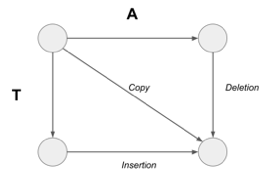(A) Copy = 0; Insertion/Deletion = 1

(B) Copy = 2; Insertion/Deletion = 1

(C) Copy = 0; Insertion = -1; Deletion = 3

(D) Copy = 2; Insertion = 1; Deletion = -1

Explanation: The copy operation should be the smallest weight of the three given - both insertions and deletions require changing the input and output in some way, and therefore should be weighted more than copy. Additionally, for this particular problem, there should be no cost difference between insertion and deletion. Both involve changing the input or output by one character. For other situations, this may change, but for this (and other similar problems you will see in this class), insertion and deletion can be considered the same.

As a note, the actual numbers do not matter all that much, as long as the logic behind them makes sense. Traditionally, copy operations are 0 weight, but can feasibly be any real number.

Q11. What path would best describe the alignment of AATGC and ATTG, using the operations and weights defined in Q10? Assume that you “start” at the upper left node (orange) and end at the lower right (blue) of the empty matrix provided.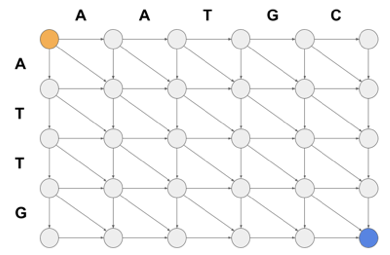(A) Copy; Insert; Insert; Insert; Insert; Delete; Delete; Delete

(B) Copy; Insert; Delete; Copy; Delete; Insert; Insert

(C) Copy; Insert; Copy; Delete; Copy; Insert

(D) Insert; Insert; Delete; Copy; Delete; Copy; Delete

Explanation: The best path is the one which minimizes the score to go from the upper left to the lower right. In option (c) there are three copy operations (weight = 0) and three insert/delete operations (weight = 1 per operation). All other paths listed have more insert/delete operations and less copy operations, and therefore are more costly.

Q12. What is the weight of the most optimal path to align these DNA strings (assuming copy = 0, and insert/delete = 1?

(A) 2

(B) 3

(C) 4

(D) 5

Explanation: The optimal path, as seen in Q11, has three copy operations and three insert/delete options. Since copy operations are weight 0, and insertions and deletions are both weight 1, the total weight for the best path would be 0 + 1 + 0 + 1 + 0 + 1 = 3. The optimal path is highlighted in the matrix below for a visual reference.